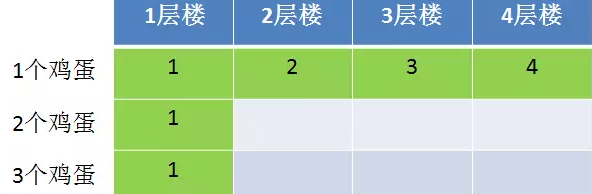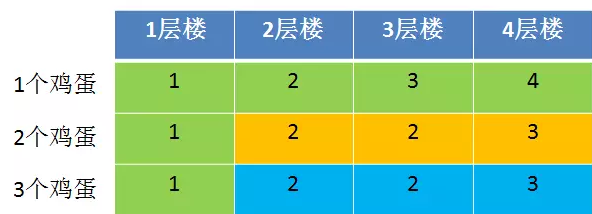# 彻底搞懂-扔鸡蛋问题-方程-动态规划

## 1、题目：

2个鸡蛋，从100层楼上往下扔，以此来测试鸡蛋的硬度，比如鸡蛋在第9层没有摔碎而在第10层摔碎了，那么鸡蛋不会摔碎的零界点就是9层，如何用最少的尝试次数，测试出鸡蛋不会摔碎的临界点？

## 2、解决思路

### 2.4、方程法：将思路逆转

so，x+(x-1)+(x-2)+...+1=100，左边多项式是各次扔鸡蛋的楼层跨度之和，假设尝试x次，所以该多项式共有x项，右边是总的楼层数100，解方程可得x=14（（x+1）*x/2=100）

## 3、动态规划解题：有M层楼N个鸡蛋，找到鸡蛋摔不碎的临界点，需要尝试几次?

1).第一个鸡蛋没碎，则剩余m-x层楼和n个鸡蛋，F(m-x,n)+1

2).第一个鸡蛋碎了，则从1到x-1层尝试，剩余鸡蛋n-1，F(x-1,n-1)+1

so，我们要求m层楼n个鸡蛋条件下，最大尝试次数的最小解，即

F(m,n)= Min(Max(F(m-x,n)+1,F(x-1,n-1)+1)) . 1<=x<=m

### 3.1、eg：3个鸡蛋，4层楼2个鸡蛋2层楼，带入状态转移方程式F(2,2)=Min( Max(F(2-x,2)+1，F(x-1,2-1)+1)) )

x可以为1和2，so：

x=1时，F(2,2)=Max(F(2-1,2)+1，F(1-1,2-1)+1))=Max(F(1,2)+1, F(0,1)+1)=Max(1+1, 0+1)=2

x=2时， F(2,2)=Max(F(2-2,2)+1，F(2-1,2-1)+1))=Max(F(0,2)+1, F(1,1)+1)=Max(0+1, 1+1)=2### 3.2、python版代码解决floor层楼仍egg个鸡蛋的问题

# 我们要求m层楼n个鸡蛋条件下，最大尝试次数的最小解，即
# F(m,n)= Min(Max(F(m-x,n)+1,F(x-1,n-1)+1)) . 1<=x<=m
import numpy as np

def get_min_floor(floors=100, eggs=2):
# 第一步，创建动态规划的备忘录，即状态转移矩阵
f = np.zeros((eggs+1, floors+1), dtype=np.int)
# 第二步，考虑边界
# part1: 先考虑eggs边界，eggs为0，则为0；eggs为1，肯定从第0层往上依次实验
for i in range(floors+1):
f[i] = 0
f[i] = i
# part2: 再考虑floor的边界，floors为0即为0，floors为1即为1
for i in range(eggs+1):
f[i] = 0
f[i] = 1
# 第三步就是状态方程了，鸡蛋从第2个开始算,楼层也从第2开始算
for egg in range(2, eggs+1):
for floor in range(2, floors+1):
# 你还有egg个鸡蛋，一共有floor层楼的子问题
# 定义一个变量来存储最终结果，找到在哪里扔能达到所扔次数最少的目标
result = 100000
# 从第drop层扔鸡蛋
for drop in range(1, floor+1):
# 碎了，剩下的问题即如何在drop-1层，用egg-1个鸡蛋寻找最优解
broken = f[egg-1][drop-1]
# 没碎，在floors-drop层，用egg个鸡蛋找最优解
unbroken = f[egg][floor-drop]
# 两种情况取最大值，因为我根本不知道鸡蛋会不会碎
condition = max(broken, unbroken)+1
# 不断和上一次结果做比较，得到最少的扔次数
result = min(condition, result)
f[egg][floor] = result
# 以上步骤在不断的往状态举证填充，到这里已经填充完毕
final_result = f[eggs][floors]
return final_result

if __name__ == '__main__':
print get_min_floor(eggs=2, floors=100)  # 输出结果14
print get_min_floor(eggs=2, floors=36)   # 输出结果8
print get_min_floor(eggs=2, floors=39)   # 输出结果9
print get_min_floor(eggs=3, floors=39)   # 输出结果6
09-234505
08-101万+07-021844
03-23383
06-182341
11-18601
09-105633
07-061万+
10-15216
09-123万+